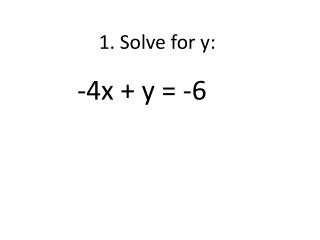DownloadDownload Presentation1. Solve for y:

# 1. Solve for y:

Télécharger la présentation## 1. Solve for y:

- - - - - - - - - - - - - - - - - - - - - - - - - - - E N D - - - - - - - - - - - - - - - - - - - - - - - - - - -
##### Presentation Transcript

1. -4x + y = -6 1. Solve for y:

2. 2y = 2x – 6 2. Solve for y:

3. 6x + 2y = 18 3. Solve for y:

4. 6x – 3y = -24 4. Solve for y:

5. 3x – 4y = -12 5. Solve for y:

6. 1. Find the point of intersection: y = 3x-4 y = -3x+2

7. 2. Find the point of intersection: y = 4x-1 y = -x+4

8. 3. Find the point of intersection:

9. 4. Find the point of intersection:

10. 1. Writeyour system of equations: Andrew’s Gym has a \$99 activation fee and costs \$35 per month. Demetri’s Gym has a \$75 activation fee and costs \$39.99 per month.

11. 2. Writeyour system of equations: Dimitriwent to Five Guys for lunch. He bought two hot dogs and three burgers for \$14. Eric also went to Five Guys for lunch. He bought four hot dogs and seven burgers for \$36.

12. 3. Writeyour system of equations: Sheyenne went to the Rodeo. She paid a \$40 admission fee and \$2 per ride. Heather went to the State Fair instead. She paid a \$30 admission fee and \$4 per ride.

13. 4. Writeyour system of equations: Michael went to Dunkin Donuts. He bought 6 donuts and 8 breakfast burritos. He spent \$9. Mario also went to Dunkin Donuts. He bought 12 donuts and 3 breakfast burritos. He spent \$8.25.

14. Now Put it All Together… Michael went to Dunkin Donuts. He bought 6 donuts and 8 breakfast burritos. He spent \$9. Mario also went to Dunkin Donuts. He bought 12 donuts and 3 breakfast burritos. He spent \$8.25. How much does one donut cost? How much does one breakfast burrito cost? Steps: • Write your equations. (What do your variables stand for?) • Solve for y if you have to. • Graph. • Find the intersection. • Answer the question(s). (Cost/Quantity/Best Deal/When same cost?)

15. 1. Graphing by HAND Put your calculator aside. Graph this equation: y = 2x + 1

16. 2. Graphing by HAND Put your calculator aside. Graph this equation: y = 2x – 1

17. 3. Graphing by HAND Put your calculator aside. Graph this equation: y = ½ x + 1

18. 4. Graphing by HAND Put your calculator aside. Graph this equation: y = ½ x – 1

19. 5. Graphing by HAND Put your calculator aside. Graph this equation: y = -½ x + 1

20. 6. Graphing by HAND Put your calculator aside. Graph this equation: y = -½ x – 1